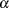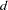The NLMIXED Procedure
 PREDICT Statement

PREDICT expression OUT=SAS-data-set <options> ;

The PREDICT statement enables you to construct predictions of an expression across all of the observations in the input data set. Any valid SAS programming expression involving the input data set variables, parameters, and random effects is valid. Predicted values are computed using the parameter estimates and empirical Bayes estimates of the random effects. Standard errors of prediction are computed using the delta method (Billingsley 1986, Cox 1998). Results are placed in an output data set that you specify with the OUT= option. Besides all variables from the input data set, the OUT= data set contains the following variables: Pred, StdErrPred, DF, tValue, Probt, Alpha, Lower, Upper. You can also add other computed quantities to this data set with the ID statement.

The following options are available in the PREDICT statement:

ALPHA=specifies the alpha level to be used in computingstatistics and intervals. The default value corresponds to the ALPHA= option in the PROC NLMIXED statement.

DER

requests that derivatives of the predicted expression with respect to all parameters be included in the OUT= data set. The variable names for the derivatives are the same as the parameter names with the prefix "Der_" appended. All of the derivatives are evaluated at the final estimates of the parameters and the empirical Bayes estimates of the random effects.

DF=specifies the degrees of freedom to be used in computingstatistics and intervals in the OUT= data set. The default value corresponds to the DF= option in the PROC NLMIXED statement.Previous Page | Next Page | Top of Page